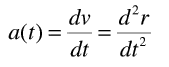# Time Dependent Acceleration

A friend of mine lead me to this math example when I asked him what math would be involved in finding the deceleration necessary to stop an already accelerated object over a certain distance. For example, a car going 50mph that must stop in 40ft.

This was the example......and I have no idea where to start. Could anyone help me interpret this? And... sorry about bugging you guys with my general lack of physics understanding.

LCKurtz
Homework Helper
Gold Member
Do you know calculus? If you want to stop with a constant deceleration a start with

$x''(t) = -a$ and integrate twice to get position as a function of a and t. Set the initial velocity to 50mph (in feet per second2), initial position x = 0 and require the velocity is 0 when x = 40. Solve for a.

Thanks, LCKurtz. And no, I don't know calculus =( And while I'm sure this is probably infinitely useful, I unfortunately don't know what it is to integrate twice. It's looking like I might be in over my head and I should look into some good calculus books.

Thanks again for the help.

LCKurtz